# Learn the Concept of Buffers in Node.JS

1
7451In the last article, we discussed the concept of event emitters in Node.js. In this article, we are going to discuss on the concept of buffers in node.js and the various operations on the buffer along with the suitable examples.

Buffers in Node.js
JavaScript works on Unicode and not on the binary data. Therefore, when we are dealing with TCP streams for files, it is necessary to handle octet streams. Node.js provides the Buffer class that provides instances with which we can store raw data in the similar way when we deal with arrays. But here the raw data storage corresponds to a raw memory allocation which is outside the V8 heap.

Buffer Class
The Buffer class is a global class in Node.js which can be accessed in an application without importing the buffer module. We can create node buffers in the following ways.

• Uninitiated Buffer of n octets: – The following is the syntax to create a buffer in Node.js n octets. Where n is a number say 100.
```var buffer = new Buffer(100);
var string = buffer.write("World!");
console.log("String Length = "+  string);```

Output: – When we execute above code, we will observe the following output.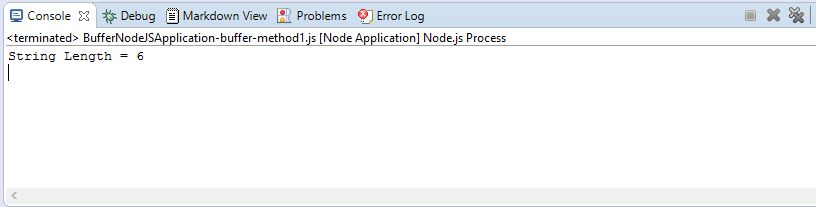• Buffer from a given array: – The following is the syntax to create a buffer from a given array.
```var buffer = new Buffer([100, 200, 300, 400, 500]);
console.log("Total array elements = "+  buffer.length);```

Output: – When we execute above code, we will observe the following output.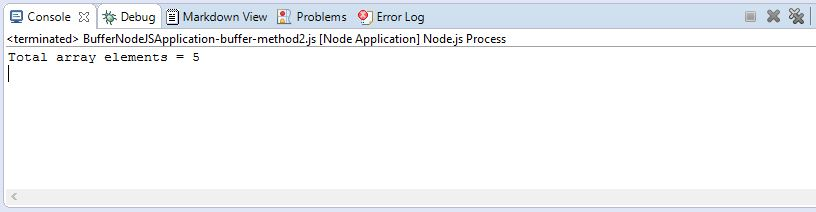• Buffer from a given string: – The following is the syntax to create a Buffer from a given string and its encoding type which is an optional field. It should be noted that “utf8” is the default encoding type. Buffer class supports the following encoding types. They are “ascii”, “utf8”, “utf16le”, “ucs2”, “base64” and “hex”.
```var buffer = new Buffer("Hello World!", "utf-8");
console.log("Contents are = "+  buffer);```

Output: – When we execute above code, we will observe the following output.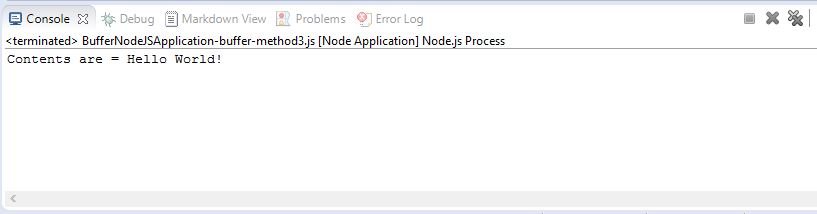Buffer Operations
The following are the various Buffer operations in Node.js.

• Writing to Buffer: – The Following is the syntax of the class method which is used to write into a Node Buffer.
`buf.write(string[, offset][, length][, encoding])`

Description: It has the following parameters
1. string – It is the actual string data to be written to buffer.
2. offset – It is the index of the buffer from where to start writing at. The default offset value is 0.
3. length − It is the number of bytes to write. The default length to buffer.length.
4. encoding – It is the encoding type to be used. The ‘utf8’ is the default encoding.

Return Value
This ‘write’ method returns the number of octets that are written. In case, there is no sufficient space in the buffer to fit the entire string, then it will write a part of the string as the return value

Example of ‘write’ method

```var buffer = new Buffer(256);
var length = buffer.write("Concept of Buffers in Node.js");

console.log("Octets written : "+  length);```

Output: When we execute above code, we will observe the following output.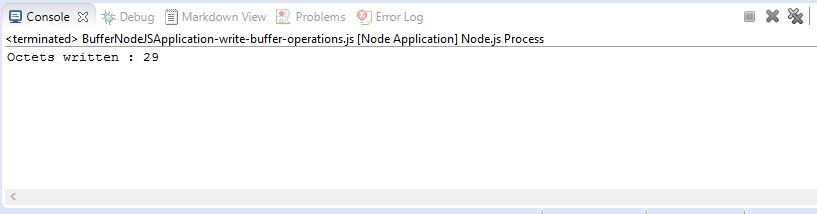• Reading from Buffers: – The Following is the syntax of the class method which is used to read from a Node Buffer.
`buf.toString([encoding][, start][, end])`

Description: – It has the following parameters
1. encoding – It is the encoding type to use. The ‘utf8’ is the default encoding type.
2. start – It is the beginning index to start reading. The defaults value is 0.
3. end – It is the end index to end reading. The default value is the complete buffer.

Return Value
The above method decodes and returns a string from buffer data which is encoded as specified in the character set encoding.

Example

```var buffer = new Buffer(26);
for (var index = 0 ; index < 26 ; index++) {
buffer[index] = index + 97;
}

console.log( buffer.toString('ascii'));
console.log( buffer.toString('ascii',23,52));
console.log( buffer.toString('utf8',0,11));
console.log( buffer.toString('utf16le',0,11));
console.log( buffer.toString('ucs2',0,11));
console.log( buffer.toString('base64',0,11));
console.log( buffer.toString('hex',0,11));
console.log( buffer.toString(undefined,0,5)); // encoding will defaults to 'utf8'```

Output: – When we execute above code, we will observe the following output.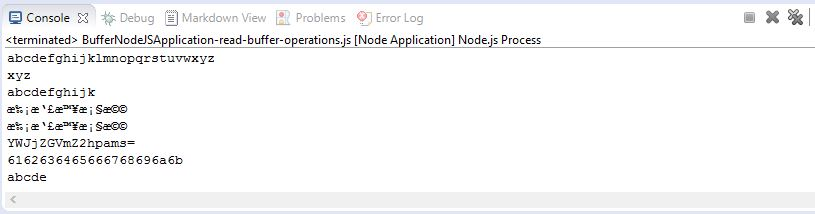• Convert Buffer to JSON: – This is the syntax of the class method which is used to convert a Node Buffer into JSON object. The returned value is a JSON-representation of the Buffer instance.
`buf.toJSON()`

Example

```var buffer = new Buffer('Hello World!');
var json = buffer.toJSON(buffer);

console.log(json);```

Output: – When we execute above code, we will observe the following output.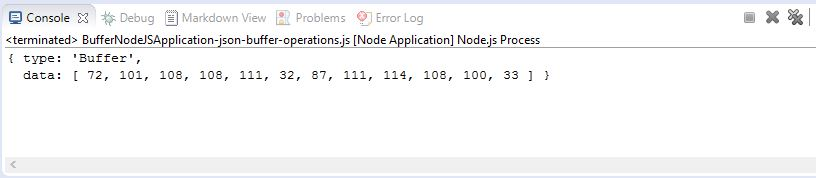• Concatenate Buffers: – This is the syntax of the class method which is used to concatenate Node buffers to a single Node Buffer.
`Buffer.concat(list[, totalLength])`

Description: – It has the following parameters
1. list – It is the array List of the Buffer objects which are to be concatenated.
2. totalLength − It is the total length of the buffers when they are concatenated.

Return Value
This method returns a Buffer instance composed of the concatenated buffers.

Example

```var buffer1 = new Buffer('Hello ');
var buffer2 = new Buffer('to the World of Node.js');
var buffer3 = Buffer.concat([buffer1,buffer2]);
console.log("buffer3 content are: " + buffer3.toString());```

Output: – When we execute above code, we will observe the following output.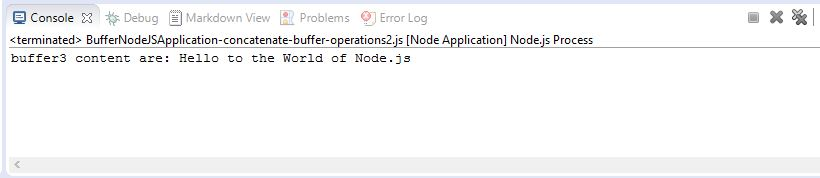• Compare Buffers: – This is the syntax of the class method which is used to compare two Node buffers.
`buf.compare(otherBuffer);`

Description: – It has the following parameters
1. otherBuffer − It is the other buffer with which the buffer will be compared.

Return Value
It returns a number in (1, 0, and -1) that indicates whether it comes before or after or it is the same as that the other Buffer in the sorting order as shown in the below example.

Example

```var buffer1 = new Buffer('Canada');
var buffer2 = new Buffer('United States');
var result = buffer1.compare(buffer2);

console.log("Value returned is : " + result);
if(result < 0) {
console.log(buffer1 +" comes before " + buffer2);
}
else if(result === 0){
console.log(buffer1 +" is same as " + buffer2);
}
else {
console.log(buffer1 +" comes after " + buffer2);
}```

Output: – When we execute above code, we will observe the following output.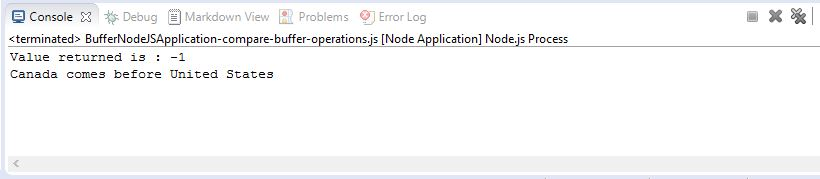• Copy Buffer: – This is the syntax of the class method which is used to copy a node buffer.
`buf.copy(targetBuffer[, targetStart][, sourceStart][, sourceEnd])`

Description: – It has the following parameters
1. targetBuffer – It is the buffer object where buffer will be copied.
2. targetStart – It is the number that specifies the target buffer start offset. It is an Optional feild. The default value is 0.
3. sourceStart − It is the number that specifies the source buffer start offset. It is an optional feild. The default value is 0.
4. sourceEnd − It is the number that specifies the source buffer end offset. It is an optional feild. The default value is buffer.length

Return Value
It has no return value. It just copies the data from a region of this buffer to a region in the target buffer.

Example

```var buffer1 = new Buffer('Hello World!');

//copy a buffer
var buffer2 = new Buffer(12);
buffer1.copy(buffer2);
console.log("buffer2 content are : " + buffer2.toString());```

Output: – When we execute above code, we will observe the following output.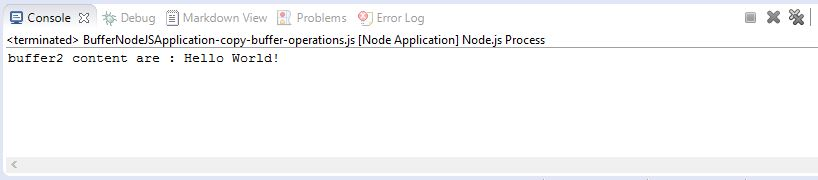• Slice Buffer: – The Following is the syntax of the class method which is used to get a sub-buffer of a node buffer.
`buf.slice([start][, end])`

Description: – It has the following parameters
1. start – It is a number that specifies the start of the buffer. It is an optional field with default value as 0.
2. end − It is a number that specifies the end of the buffer. It is an optional field with default value as buffer.length

Return Value
It returns a new buffer which has the same reference memory as the old one, but the offset are cropped between the start (defaults to 0) and end (defaults to buffer.length) indexes. It should be noted that the negative indexes start from the end of the buffer.

Example

```var buffer1 = new Buffer('Czechoslovakia');

//slicing a buffer
var buffer2 = buffer1.slice(6,15);
console.log("buffer2 content are : " + buffer2.toString());```

Output: When we execute above code, we will observe the following output.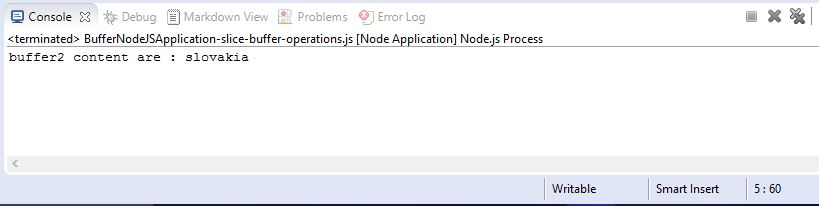• Buffer Length: – This is the syntax of the class method which is used to get a size of a node buffer in bytes.
`buf.length;`

Return Value
It returns the size of a buffer in bytes.

Example

```var buffer = new Buffer('Eduonix tutorials');

console.log("The length of buffer is : " + buffer.length);```

Output: – When we execute above code, we will observe the following output.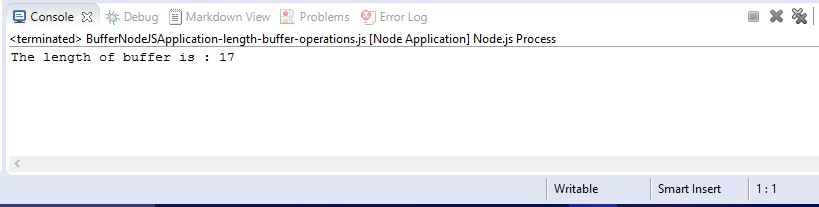Class Methods Reference
The following are the few references of Buffers module available in Node.js.

 S No. Syntax Description 1. new Buffer(size) This syntax is used to allocate a new buffer of size in octets. It should be noted that the size should not exceed the Maximum length, if exceeded then it will throw a RangeError. 2. new Buffer(buffer) This syntax is used to copy the buffer data which is passed as a parameter onto a new Buffer instance. 3. new Buffer(str[, encoding]) This syntax is used to allocate a new buffer containing the given str. encoding defaults to ‘utf8’. 4. buf.length This syntax is used to return the size of the buffer in bytes. It should be noted that it is not necessarily the size of the contents. Actually, the length refers to the amount of memory allocated for the buffer object. 5. buf.write (string[, offset][, length][, encoding]) This syntax is used to write a string to the buffer at offset using the given encoding. The default value of the offset is 0. The default value of encoding is ‘utf8’. The length is the number of bytes that we want to write. It returns the number of octets that we have written.

Source code for the concept of Buffer in Nodejs

Conclusion: –
In this article, we have covered the ‘Buffer’ class the various operations associated with the ‘Buffer’ class in Node.js along with the suitable examples which are required by a beginner developer to work.

#### 1 COMMENT

1.Mike Traffanstead

The Buffer constructors have been deprecated since v6.0.0 and should not be used. You should use Buffer.from() and Buffer.alloc() instead.